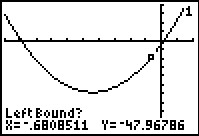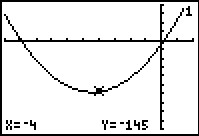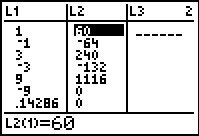# Activities

••• ##### Subject Area

• Math: Algebra II: Polynomials

• ##### Author9-12

30 Minutes

• ##### Device
• TI-83 Plus Family
• TI-84 Plus
• TI-84 Plus Silver Edition
•TI-84 Plus C Silver Edition
•TI-84 Plus CE
• ##### Report an Issue#### Activity Overview

Students will use the Rational Zero Theorem to find all rational zeros of a polynomial.

#### Key Steps

•Students will investigate the Rational Zero Theorem. They will identify the number of real roots by graphing and use the graph to approximate real roots.

•Students will then use the Rational Zero Theorem to find all the potential rational zeros of the function. Using the calculator, they will use a list to calculate the y value for each of the potential zeros to find the actual rational zeros.

•Students will then use synthetic division to find other zeros, and verify the answers with the calculator. By the end of this lesson, students will identify rational and real roots of polynomials, and discuss why polynomials would have no rational or real roots.